August 29, 2017

## Learning Objectives

• Recap multiple regression
• Fit, forecast, residuals in multiple regressions
• Seasonal dummies
• Trend
• Residuals and residual autocorrelation
• Regressions in matrix form (H hat matrix)
• Nonlinear regressions and splides
• Correlation and causation

## Multiple regression

In multiple regression there is one variable to be forecast and several predictor variables.

• The credit score is used to determine if a customer will be given a loan or not. The credit score could be predicted from other variables. This is an example of cross-sectional data where we want to predict the value of the credit score variable using the values of other variables.

• We want to forecast the value of future beer production, but there are no other variables available for predictors. Instead, with time series data, we could use the number of quarters since the start of the series, or the quarter of the year corresponding to each observation as a predictor variable.

## Introduction to multiple regression

The general form of a multiple regression is

$y_{i} = \beta_{0} + \beta_{1} x_{1,i} + \beta_{2} x_{2,i} + \cdots + \beta_{k} x_{k,i} + e_{i},$

where $$y_{i}$$ is the variable to be forecast and $$x_{1,i},\dots,x_{k,i}$$ are the $$k$$ predictor variables.

The coefficients $$\beta_{1},\dots,\beta_{k}$$ measure the effect of each predictor after taking account of the effect of all other predictors in the model.

Thus, the coefficients measure the marginal effects of the predictor variables.

## Introduction to multiple regression

For forecasting we require the following assumptions for the errors $$(e_{1},\dots,e_{N})$$:

• the errors have mean zero;
• the errors are uncorrelated with each other;
• the errors are uncorrelated with each predictor $$x_{j,i}$$.

It is also useful to have the errors normally distributed with constant variance in order to produce prediction intervals, but this is not necessary for forecasting.

## Example: credit scores

#The panel.hist function is defined in help(pairs).
pairs(credit[,-(4:5)], diag.panel=panel.hist)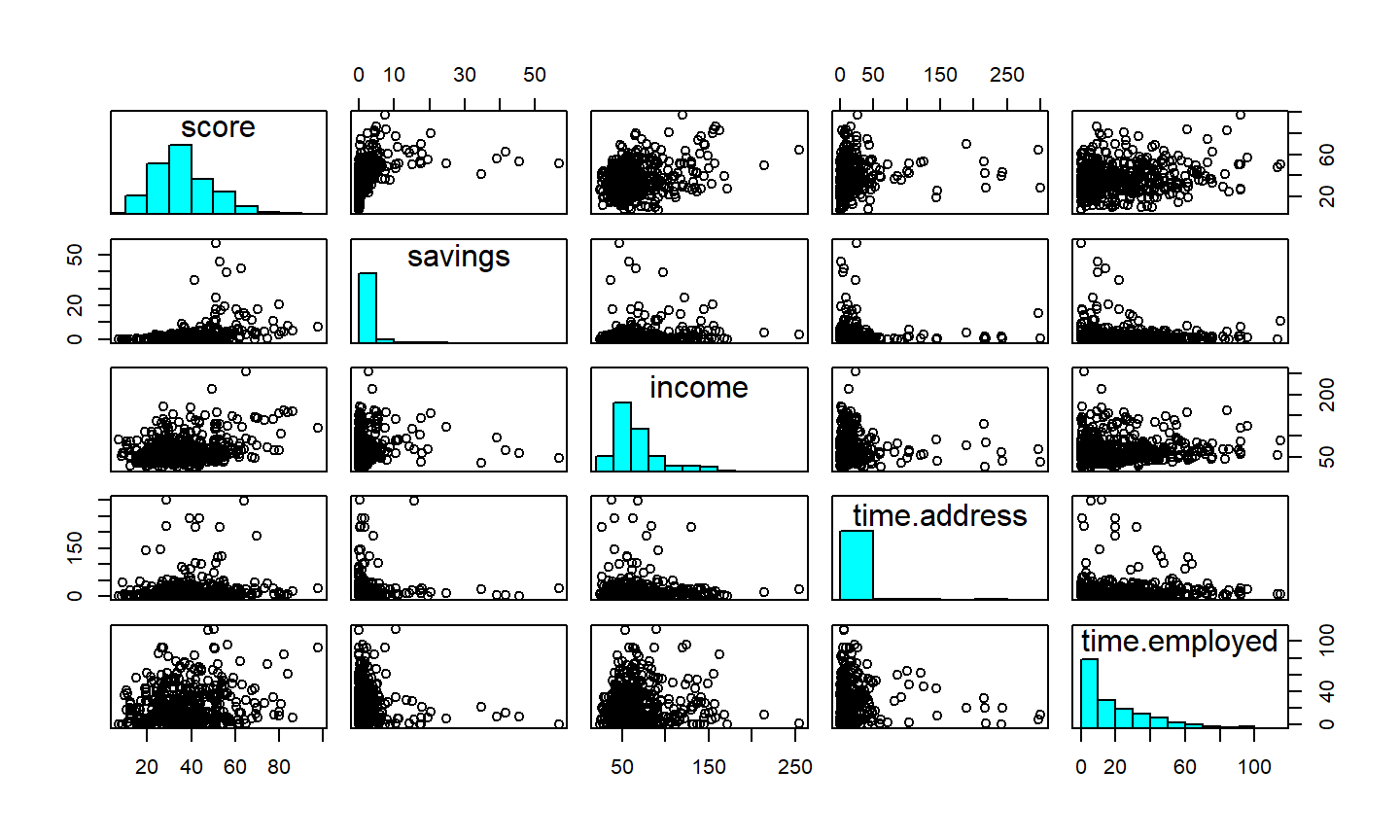The predictors are all highly skewed and the few outlying observations are making it hard to see what is going on in the bulk of the data.

We cannot take logarithms of the predictors because they contain some zeros. Instead, we use the transformation $$\log(x+1)$$: zeros on the original scale remaining zeros on the transformed scale.

creditlog <- data.frame(score=credit$score, log.savings=log(credit$savings+1),
log.income=log(credit$income+1), log.address=log(credit$time.address+1),
log.employed=log(credit$time.employed+1), fte=credit$fte, single=credit$single) pairs(creditlog[,1:5],diag.panel=panel.hist)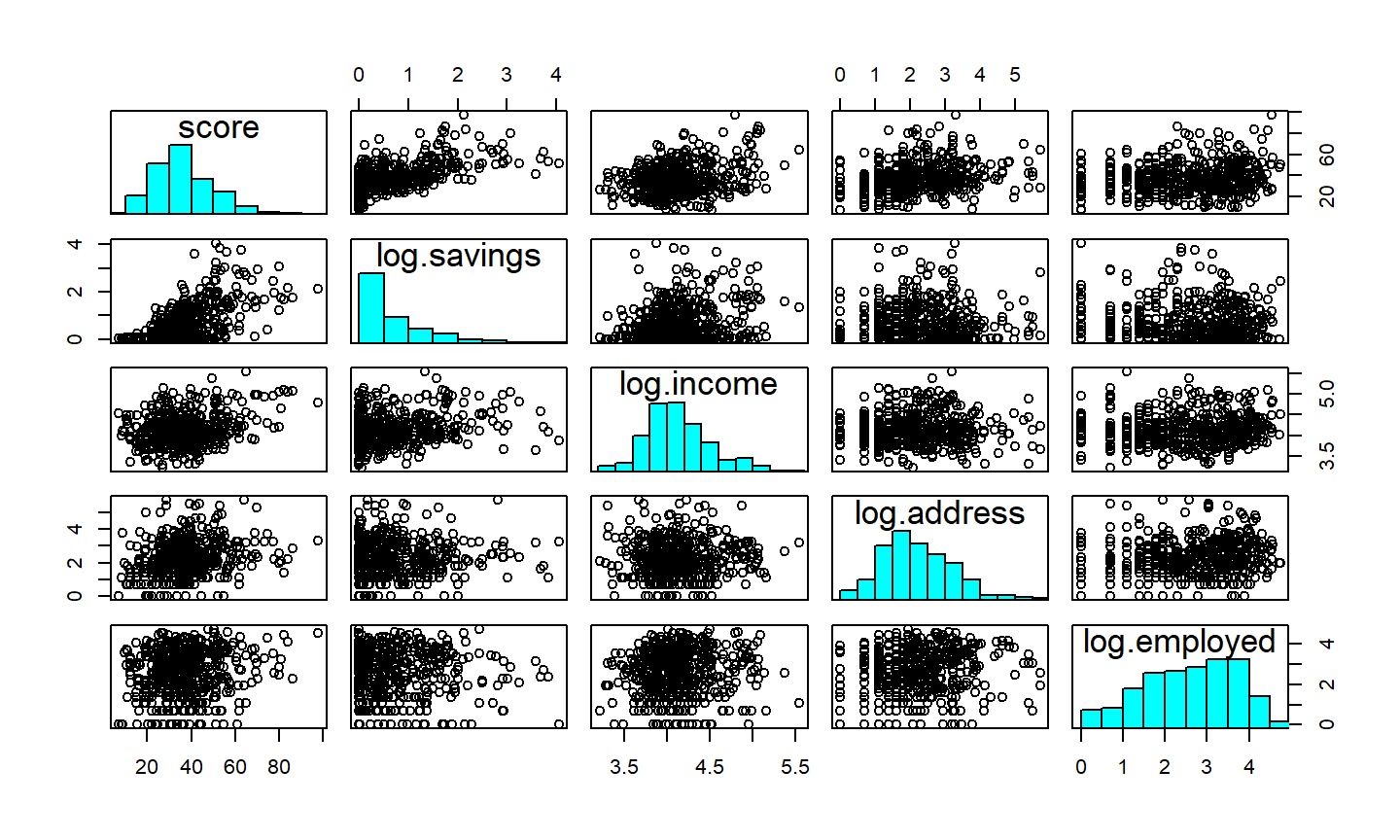## Estimation of the model The values of the coefficients $$\beta_{0},\dots,\beta_{k}$$ are obtained by finding the minimum sum of squares of the errors. That is, we find the values of $$\beta_{0},\dots,\beta_{k}$$ which minimize $\sum_{i=1}^N e_{i}^2 = \sum_{i=1}^N (y_{i} - \beta_{0} - \beta_{1}x_{1,i} - \cdots - \beta_{k} x_{k,i})^2.$ This is called least squares estimation because it gives the least value of the sum of squared errors. Finding the best estimates of the coefficients is often called fitting the model to the data. We refer to the estimated coefficients using the notation $$\hat\beta_{0},\dots,\hat\beta_{k}$$. ## Estimation of the model summary(fit)$coef
##               Estimate Std. Error     t value     Pr(>|t|)
## (Intercept)  -0.218631  5.2308536 -0.04179643 9.666778e-01
## log.savings  10.352557  0.6124476 16.90357898 6.192642e-51
## log.income    5.052122  1.2579262  4.01623095 6.831722e-05
## log.address   2.666642  0.4344902  6.13740485 1.720601e-09
## log.employed  1.313810  0.4094249  3.20891643 1.418772e-03

Run summary(fit) in R to see full output.

## Fit, forecast, residuals

Predictions of $$y$$ can be calculated by ignoring the error in the regression equation:

$\hat{y} = \hat\beta_{0} + \hat\beta_{1} x_{1} + \hat\beta_{2} x_{2} + \cdots + \hat\beta_{k} x_{k}.$

Plugging in values of $$x_{1},\dots,x_{k}$$ into the right hand side of this equation gives a prediction of $$y$$ for that combination of predictors.

When this calculation is done using values of the predictors from the data that were used to estimate the model, we call the $$\hat{y}$$ the fitted values. These are "predictions" of the data used in estimating the model. They are not genuine forecasts as the actual value of $$y$$ for that set of predictors was used in estimating the model, and so the value of $$\hat{y}$$ is affected by the true value of $$y$$.

## Fit, forecast, residuals

When the values of $$x_{1},\dots,x_{k}$$ are new values (i.e., not part of the data that were used to estimate the model), the resulting value of $$\hat{y}$$ is a genuine forecast.

The difference between the $$y$$ observations and the fitted values are the residuals:

$e_i = y_i - \hat{y}_i = y_i - \hat\beta_{0} - \hat\beta_{1} x_{1} - \hat\beta_{2} x_{2} - \cdots - \hat\beta_{k} x_{k}.$

The residuals have zero mean and are uncorrelated with any of the predictors.

## $$R^2$$: the coefficient of determination

The $$R^2$$ value is the square of the correlation between the actual values and the predicted values.

The value of $$R^2$$ can also be calculated as the proportion of variation in the forecast variable that is explained by the regression model:

$R^2 = \frac{\sum(\hat{y}_{i} - \bar{y})^2}{\sum(y_{i}-\bar{y})^2}$

In this case, $$R^2=0.47$$, so about half of the variation in the scores can be predicted using the model. Thus, the model is not really sufficient to replace a more detailed approach to credit scoring, but it might be helpful in filtering out customers who will get a very low score.

plot(fitted(fit), creditlogscore, las = 1, col = "blue", pch = "+", ylab="Score", xlab="Predicted score")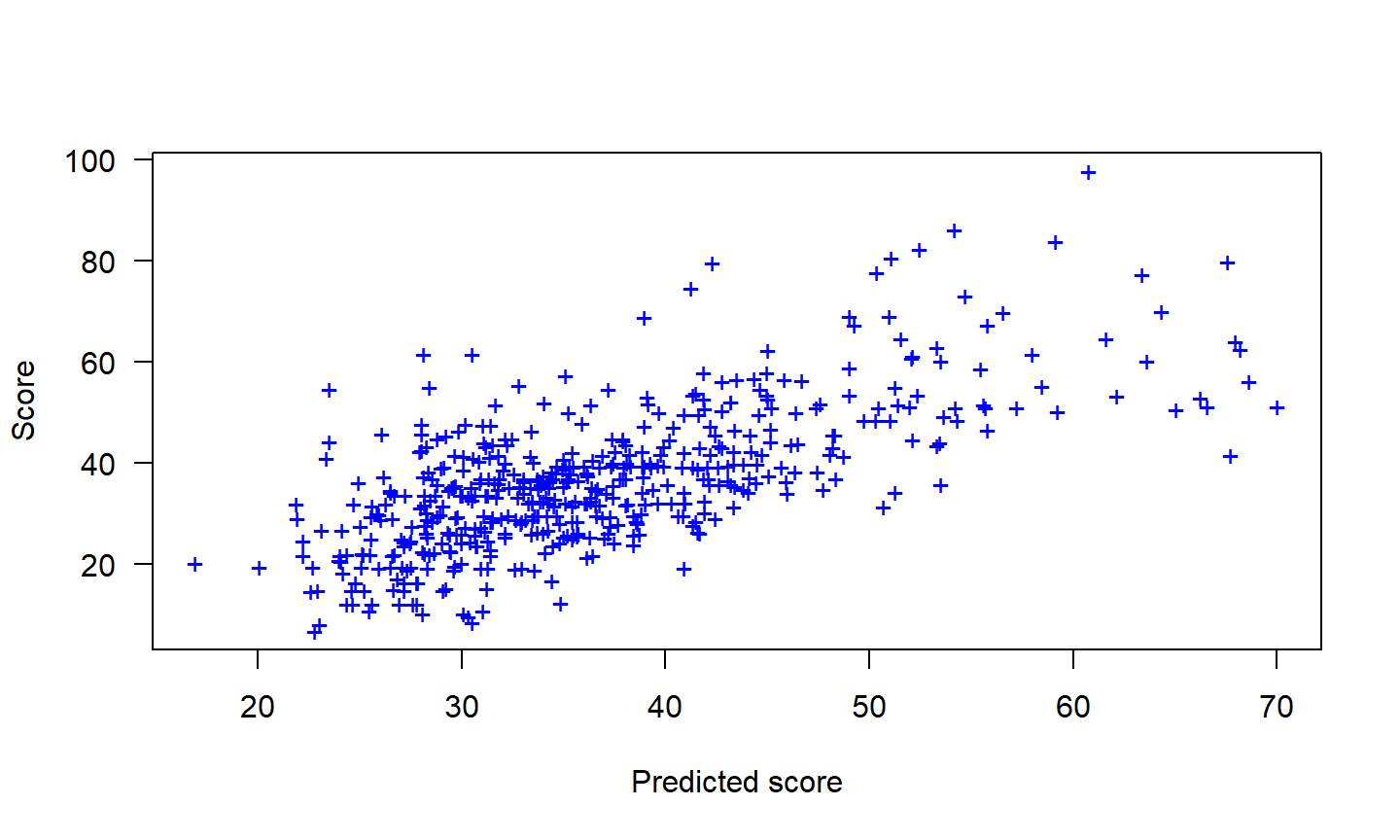## Dummy variables A predictor could be a categorical variable taking only two values (e.g., "yes" and "no"). This situation can still be handled within the framework of multiple regression models by creating a "dummy variable" taking value 1 corresponding to "yes" and 0 corresponding to "no". A dummy variable is also known as an "indicator variable". If there are more than two categories, then the variable can be coded using several dummy variables (one fewer than the total number of categories). ## Seasonal dummy variables For example, suppose we are forecasting daily electricity demand and we want to account for the day of the week as a predictor. Then the following dummy variables can be created. Day D1 D2 D3 D4 D5 D6 Monday 1 0 0 0 0 0 Tuesday 0 1 0 0 0 0 Wednesday 0 0 1 0 0 0 Thursday 0 0 0 1 0 0 Friday 0 0 0 0 1 0 Saturday 0 0 0 0 0 1 ## Seasonal dummy variables Day D1 D2 D3 D4 D5 D6 Sunday 0 0 0 0 0 0 Monday 1 0 0 0 0 0 Tuesday 0 1 0 0 0 0 Wednesday 0 0 1 0 0 0 Thursday 0 0 0 1 0 0 Friday 0 0 0 0 1 0 Saturday 0 0 0 0 0 1 Sunday 0 0 0 0 0 0 Monday 1 0 0 0 0 0 Tuesday 0 1 0 0 0 0 $$\vdots$$ $$\vdots$$ $$\vdots$$ $$\vdots$$ $$\vdots$$ $$\vdots$$ $$\vdots$$ Notice that only six dummy variables are needed to code seven categories. That is because the seventh category (in this case Sunday) is specified when the dummy variables are all set to zero. Many beginners will try to add a seventh dummy variable for the seventh category (dummy variable trap). The interpretation of each of the coefficients associated with the dummy variables is that it is a measure of the effect of that category relative to the omitted category. In the above example, the coefficient associated with Monday will measure the effect of Monday compared to Sunday on the forecast variable. Other uses of dummy variables: outliers. If there is an outlier in the data, rather than omit it, you can use a dummy variable to remove its effect. In this case, the dummy variable takes value one for that observation and zero everywhere else. ## Trend A linear trend is easily accounted for by including the predictor $$x_{1,t}=t$$. A piecewise linear trend with a bend at time $$\tau$$ can be specified by including the following predictors in the model. \begin{align*} x_{1,t} & = t \\ x_{2,t} & = \left\{ \begin{array}{ll} 0 & t \lt \tau\\ (t-\tau) & t \ge \tau \end{array}\right. \end{align*} If the associated coefficients of $$x_{1,t}$$ and $$x_{2,t}$$ are $$\beta_1$$ and $$\beta_2$$, then $$\beta_1$$ gives the slope of the trend before time $$\tau$$, while the slope of the line after time $$\tau$$ is given by $$\beta_1+\beta_2$$. ## Ex post and ex ante forecasting Ex ante forecasts are those that are made using only the information that is available in advance, while ex post forecasts are those that are made using later information on the predictors. Normally, we cannot use future values of the predictor variables when producing ex ante forecasts because their values will not be known in advance. However, the special predictors introduced in this section are all known in advance, as they are based on calendar variables (e.g., seasonal dummy variables or public holiday indicators) or deterministic functions of time. In such cases, there is no difference betweeen ex ante and ex post forecasts. ## Example: Australian quarterly beer production Regression model with a linear trend and quarterly dummy variables: $y_{t} = \beta_{0} + \beta_{1} t + \beta_{2}d_{2,t} + \beta_3 d_{3,t} + \beta_4 d_{4,t} + e_{t},$ where $$d_{i,t} = 1$$ if $$t$$ is in quarter $$i$$ and 0 otherwise. The first quarter variable has been omitted, so the coefficients associated with the other quarters are measures of the difference between those quarters and the first quarter. ## ## Call: ## tslm(formula = beer2 ~ trend + season) ## ## Residuals: ## Min 1Q Median 3Q Max ## -44.024 -8.390 0.249 8.619 23.320 ## ## Coefficients: ## Estimate Std. Error t value Pr(>|t|) ## (Intercept) 441.8141 4.5338 97.449 < 2e-16 *** ## trend -0.3820 0.1078 -3.544 0.000854 *** ## season2 -34.0466 4.9174 -6.924 7.18e-09 *** ## season3 -18.0931 4.9209 -3.677 0.000568 *** ## season4 76.0746 4.9268 15.441 < 2e-16 *** ## --- ## Signif. codes: 0 '***' 0.001 '**' 0.01 '*' 0.05 '.' 0.1 ' ' 1 ## ## Residual standard error: 13.01 on 51 degrees of freedom ## Multiple R-squared: 0.921, Adjusted R-squared: 0.9149 ## F-statistic: 148.7 on 4 and 51 DF, p-value: < 2.2e-16 plot(beer2, xlab="Year", ylab="", main="Quarterly Beer Production") lines(fitted(fit), col=2) legend("topright", lty=1, col=c(1,2), legend = c("Actual", "Predicted"))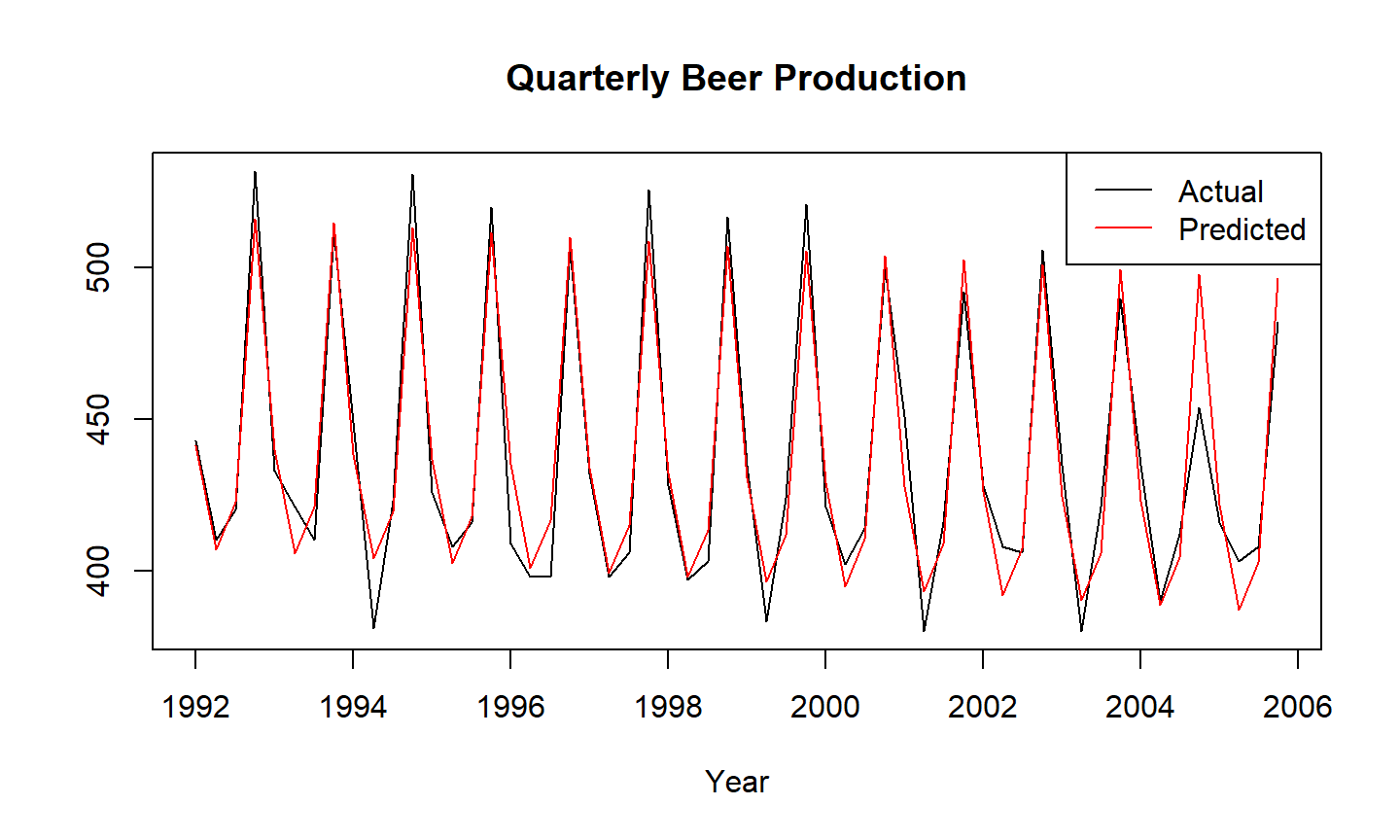plot(fitted(fit), beer2, xy.lines=FALSE, xy.labels=FALSE, xlab="Predicted values", ylab="Actual values", main="Quarterly Beer Production") abline(0, 1, col="gray")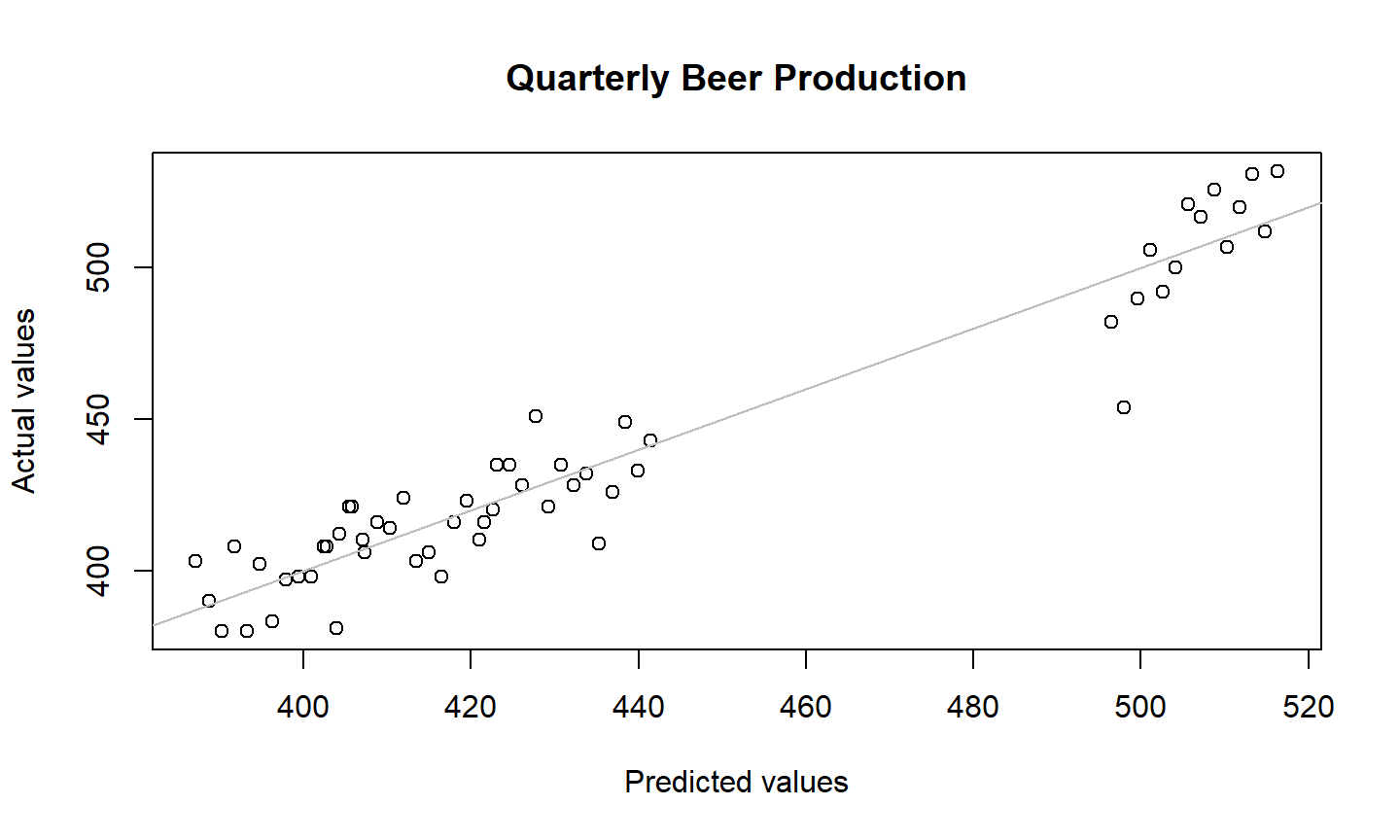fcast <- forecast(fit) plot(fcast, main="Forecasts of beer production using linear regression")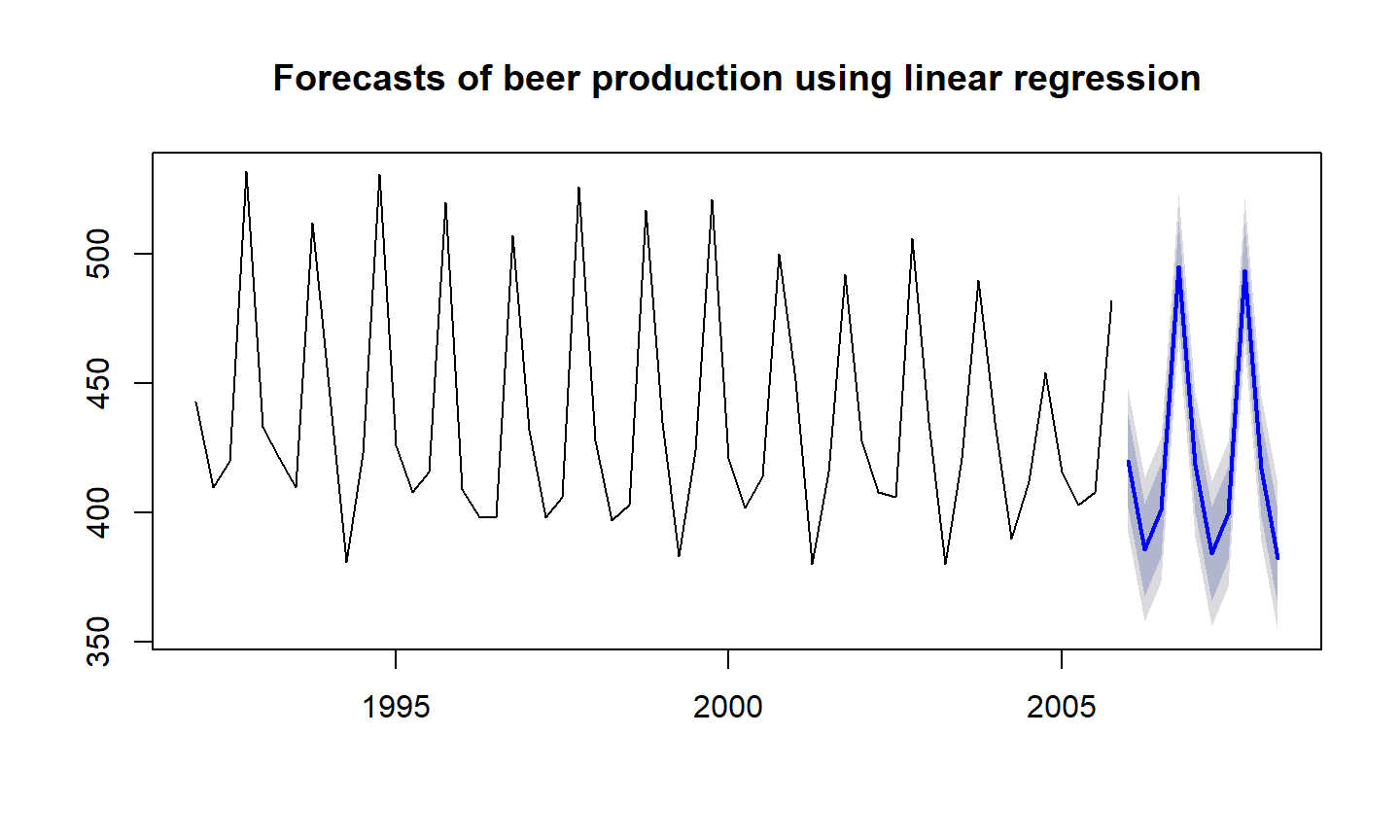## Intervention variables It is often necessary to model interventions that may have affected the variable to be forecast. For example, competitor activity, advertising expenditure, industrial action, and so on, can all have an effect. When the effect lasts only for one period, we use a dummy variable for handling an outlier (spike). If an intervention causes a level shift (i.e., the value of the series changes suddenly and permanently from the time of intervention), then we use a step variable. A step variable takes value zero before the intervention and one from the time of intervention onwards. Another form of permanent effect is a change of slope. Here the intervention is handled using a piecewise linear trend as discussed earlier (where $$\tau$$ is the time of intervention). ## Trading days The number of trading days in a month can vary considerably and can have a substantial effect on sales data. To allow for this, the number of trading days in each month can be included as a predictor. An alternative that allows for the effects of different days of the week has the following predictors. \begin{align*} x_{1} &= \text{# Mondays in month;} \\ x_{2} &= \text{# Tuesdays in month;} \\ & \vdots \\ x_{7} &= \text{# Sundays in month.} \end{align*} ## Distributed lags It is often useful to include advertising expenditure as a predictor. However, since the effect of advertising can last beyond the actual campaign, we need to include lagged values of advertising expenditure. So the following predictors may be used. \begin{align*} x_{1} &= \text{advertising for previous month;} \\ x_{2} &= \text{advertising for two months previously;} \\ &\vdots \\ x_{m} &= \text{advertising for m months previously.} \end{align*} It is common to require the coefficients to decrease as the lag increases. ## Selecting predictors When there are many possible predictors, we need some strategy to select the best predictors to use in a regression model. Not recommended: • plot the forecast variable against a particular predictor and if it shows no noticeable relationship, drop it. • do a multiple linear regression on all the predictors and disregard all variables whose p-values are greater than 0.05. Instead, use a measure of predictive accuracy: Adjusted $$R^2$$, Cross-validation, Akaike's Information Criterion, Corrected Akaike's Information Criterion, Schwarz Bayesian Information Criterion. ## Adjusted $$R^2$$ $$R^2$$ is not a good measure of the predictive ability of a model. In a model which produces forecasts that are exactly 20% of the actual values, the $$R^2$$ = 1 (indicating perfect correlation), but the forecasts are not close to the actual values. Also, $$R^2$$ does not allow for degrees of freedom. Adding any variable tends to increase the value of $$R^2$$, even if that variable is irrelevant. An equivalent idea is to select the model which gives the minimum sum of squared errors (SSE): $\text{SSE} = \sum_{i=1}^N e_{i}^2.$ Minimizing the SSE is equivalent to maximizing $$R^2$$ and will always choose the model with the most variables, and so is not a valid way of selecting predictors. An alternative, designed to overcome these problems, is the adjusted $$R^2$$: $\bar{R}^2 = 1-(1-R^2)\frac{N-1}{N-k-1},$ where $$N$$ is the number of observations and $$k$$ is the number of predictors. This is an improvement on $$R^2$$ as it will no longer increase with each added predictor. Maximizing $$\bar{R}^2$$ is equivalent to minimizing the following estimate of the variance of the forecast errors: $\hat{\sigma}^2 = \frac{\text{SSE}}{N-k-1}.$ Maximizing $$\bar{R}^2$$ works quite well as a method of selecting predictors, although it does tend to err on the side of selecting too many predictors. ## Cross-validation Cross-validation is a very useful way of determining the predictive ability of a model. In general, leave-one-out cross-validation for regression can be carried out using the following steps. 1. Remove observation $$i$$ from the data set, and fit the model using the remaining data. Then compute the error ($$e_{i}^*=y_{i}-\hat{y}_{i}$$) for the omitted observation. (This is not the same as the residual because the $$i$$th observation was not used in estimating the value of $$\hat{y}_{i}$$.) 2. Repeat step 1 for $$i=1,\dots,N$$. 3. Compute the MSE from $$e_1^*,\dots,e_{N}^*$$. We shall call this the CV. Under this criterion, the best model is the one with the smallest value of CV. ## Akaike's Information Criterion A closely-related method is Akaike's Information Criterion, which we define as $\text{AIC} = N\log\left(\frac{\text{SSE}}{N}\right) + 2(k+2),$ where $$N$$ is the number of observations used for estimation and $$k$$ is the number of predictors in the model. The $$k+2$$ part of the equation occurs because there are $$k+2$$ parameters in the model — the $$k$$ coefficients for the predictors, the intercept and the variance of the residuals. The model with the minimum value of the AIC is often the best model for forecasting. For large values of $$N$$, minimizing the AIC is equivalent to minimizing the CV value. ## Corrected Akaike's IC For small values of $$N$$, the AIC tends to select too many predictors, and so a bias-corrected version of the AIC has been developed: $\text{AIC}_{\text{c}} = \text{AIC} + \frac{2(k+2)(k+3)}{N-k-3}.$ As with the AIC, the AICc should be minimized. ## Schwarz Bayesian IC $\text{BIC} = N\log\left(\frac{\text{SSE}}{N}\right) + (k+2)\log(N).$ The model chosen by BIC is either the same as that chosen by AIC, or one with fewer terms. This is because BIC penalizes the SSE more heavily than the AIC. Many statisticians like to use BIC because it has the feature that if there is a true underlying model, then with enough data the BIC will select that model. However, in reality there is rarely if ever a true underlying model, and even if there was a true underlying model, selecting that model will not necessarily give the best forecasts (because the parameter estimates may not be accurate). To obtain all these measures in R, use CV(fit) ## CV AIC AICc BIC AdjR2 ## 186.985651 294.097630 295.811916 306.249740 0.914856 On a side note: x <- CV(fit) (round(x, digits=2)) ## CV AIC AICc BIC AdjR2 ## 186.99 294.10 295.81 306.25 0.91 ## Example: credit scores (continued) In the credit scores regression model we used four predictors. Now we can check if all four predictors are actually useful, or whether we can drop one or more of them. With four predictors, there are $$2^4 = 16$$ possible models. Part of the results is summarized in the table below. An $$X$$ indicates that the variable was included in the model. Savings Income Address Employ. CV AIC AICc BIC Adj R2 X X X X 104.7 2325.8 2325.9 2351.1 0.4658 X X X 106.5 2334.1 2334.2 2355.1 0.4558 X X X 107.7 2339.8 2339.9 2360.9 0.4495 X X 109.7 2349.3 2349.3 2366.1 0.4379 X X X 112.2 2360.4 2360.6 2381.5 0.4263 X X 115.1 2373.4 2373.5 2390.3 0.4101 X X 116.1 2377.7 2377.8 2394.6 0.4050 X 119.5 2392.1 2392.2 2404.8 0.3864 ## Best subset regression Where possible, all potential regression models can be fitted and the best one selected based on one of the measures discussed here. This is known as "best subsets" regression or "all possible subsets" regression. It is recommended that one of CV, AIC or AICc be used for this purpose. If the value of $$N$$ is large enough, they will all lead to the same model. Most software packages will at least produce AIC, although CV and AICc will be more accurate for smaller values of $$N$$. While $$\bar{R}^2$$ is very widely used, and has been around longer than the other measures, its tendency to select too many variables makes it less suitable for forecasting than either CV, AIC or AICc. Also, the tendency of BIC to select too few variables makes it less suitable for forecasting than either CV, AIC or AICc. ## Stepwise regression If there are a large number of predictors, it is not possible to fit all possible models. An approach that works quite well is backwards stepwise regression: • Start with the model containing all potential predictors. • Try subtracting one predictor at a time. Keep the model if it improves the measure of predictive accuracy. • Iterate until no further improvement. It is important to realize that a stepwise approach is not guaranteed to lead to the best possible model. But it almost always leads to a good model. ## Residual diagnostics The residuals from a regression model are calculated as the difference between the actual values and the fitted values: $$e_{i} = y_{i}-\hat{y}_{i}$$. Each residual is the unpredictable component of the associated observation. After selecting the regression variables and fitting a regression model, it is necessary to plot the residuals to check that the assumptions of the model have been satisfied. There are a series of plots that should be produced in order to check different aspects of the fitted model and the underlying assumptions. ## Scatterplots of residuals against predictors Do a scatterplot of the residuals against each predictor in the model and against any predictions not in the model (possibly in a nonlinear form). If these scatterplots show a pattern, then the model will need to be modified accordingly. fit <- lm(score ~ log.savings + log.income + log.address + log.employed, data=creditlog) par(mfrow=c(2,2)) plot(creditloglog.savings,residuals(fit),xlab="log(savings)")
plot(creditlog$log.income,residuals(fit),xlab="log(income)") plot(creditlog$log.address,residuals(fit),xlab="log(address)")
plot(creditlog$log.employed,residuals(fit),xlab="log(employed)") ## Residuals versus predictors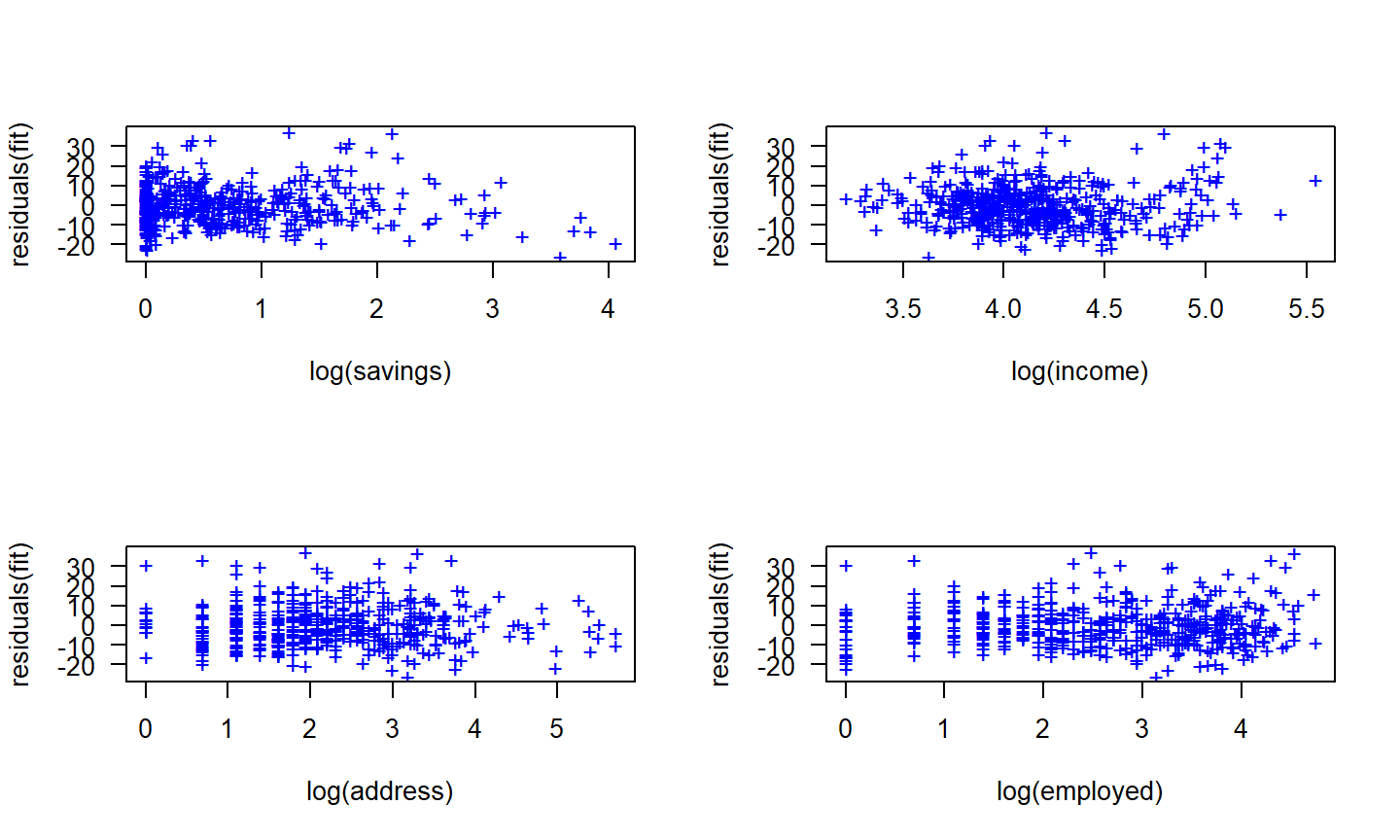## Scatterplot of residuals against fitted values A plot of the residuals against the fitted values should show no pattern. If a pattern is observed, there may be "heteroscedasticity" in the errors. That is, the variance of the residuals may not be constant. To overcome this problem, a transformation of the forecast variable (such as a logarithm or square root) may be required. plot(fitted(fit), residuals(fit), xlab="Predicted scores", ylab="Residuals") ## Residuals versus fitted values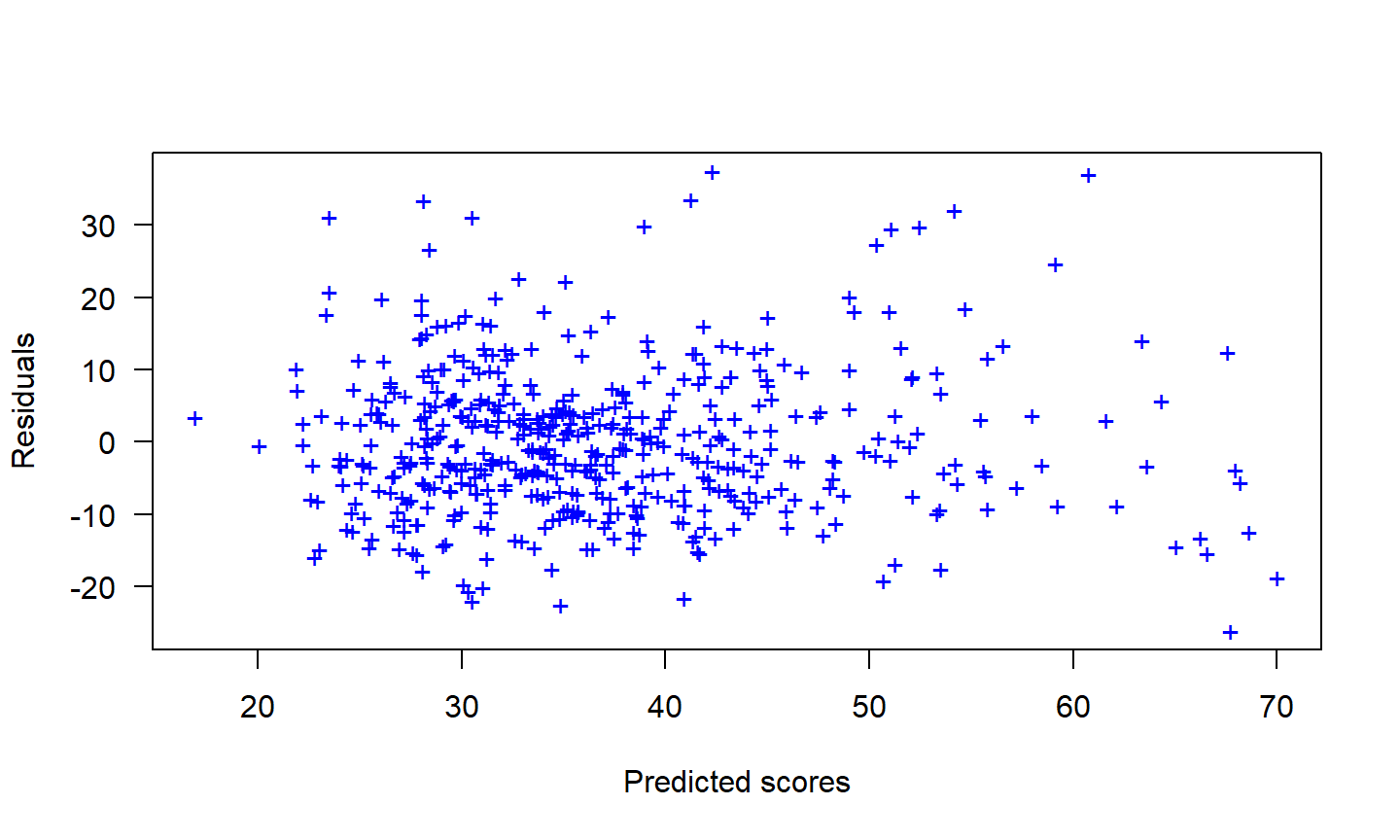## Autocorrelation in the residuals When the data are a time series, you should look at an ACF plot of the residuals. This will reveal if there is any autocorrelation in the residuals (suggesting that there is information that has not been accounted for in the model). fit <- tslm(beer2 ~ trend + season) res <- residuals(fit) par(mfrow=c(1,2)) plot(res, ylab="Residuals",xlab="Year") Acf(res, main="ACF of residuals")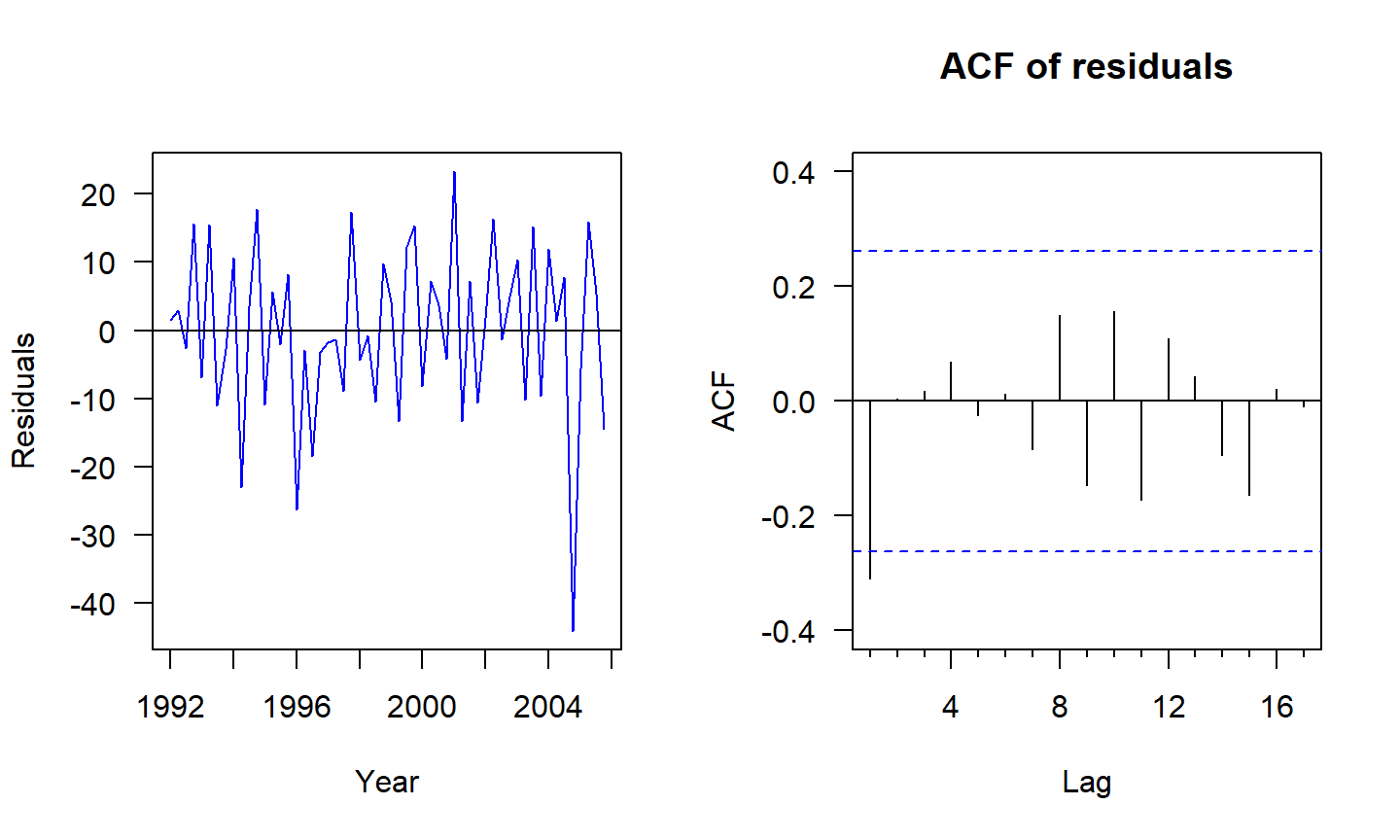There is an outlier in the residuals (2004:Q4) which suggests there was something unusual happening in that quarter.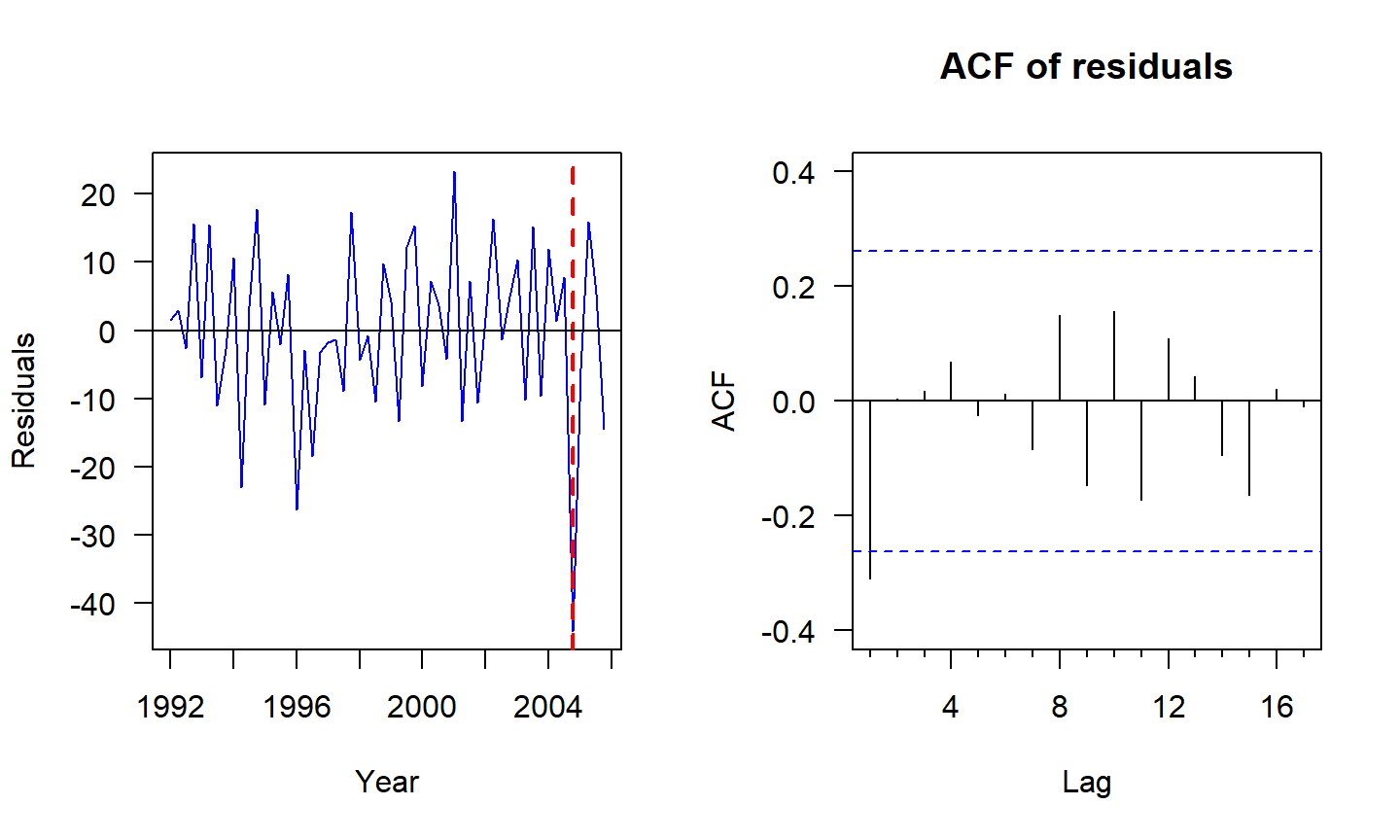There is an outlier in the residuals (2004:Q4) which suggests there was something unusual happening in that quarter. The Durbin-Watson test is used to test the hypothesis that there is no lag one autocorrelation in the residuals. If there is no autocorrelation, the DW distribution is symmetric around 2. # It is recommended that the two-sided test always be used # to check for negative as well as positive autocorrelation dwtest(fit, alt="two.sided") ## ## Durbin-Watson test ## ## data: fit ## DW = 2.5951, p-value = 0.02764 ## alternative hypothesis: true autocorrelation is not 0 There is some information remaining in the residuals that can be exploited to obtain better forecasts. The forecasts from the current model are still unbiased, but will have larger prediction intervals than they need to. The Breusch-Godfrey test is designed to look for significant higher-lag autocorrelations. # Test for autocorrelations up to lag 5. bgtest(fit,5) ## ## Breusch-Godfrey test for serial correlation of order up to 5 ## ## data: fit ## LM test = 6.4329, df = 5, p-value = 0.2663 ## Histogram of residuals It is a good idea to check if the residuals are normally distributed. As explained earlier, this is not essential for forecasting, but it does make the calculation of prediction intervals much easier. hist(res, breaks="FD", xlab="Residuals", main="Histogram of residuals", ylim=c(0,22)) x <- -50:50 lines(x, 560*dnorm(x,0,sd(res)),col=2) ## Histogram of residuals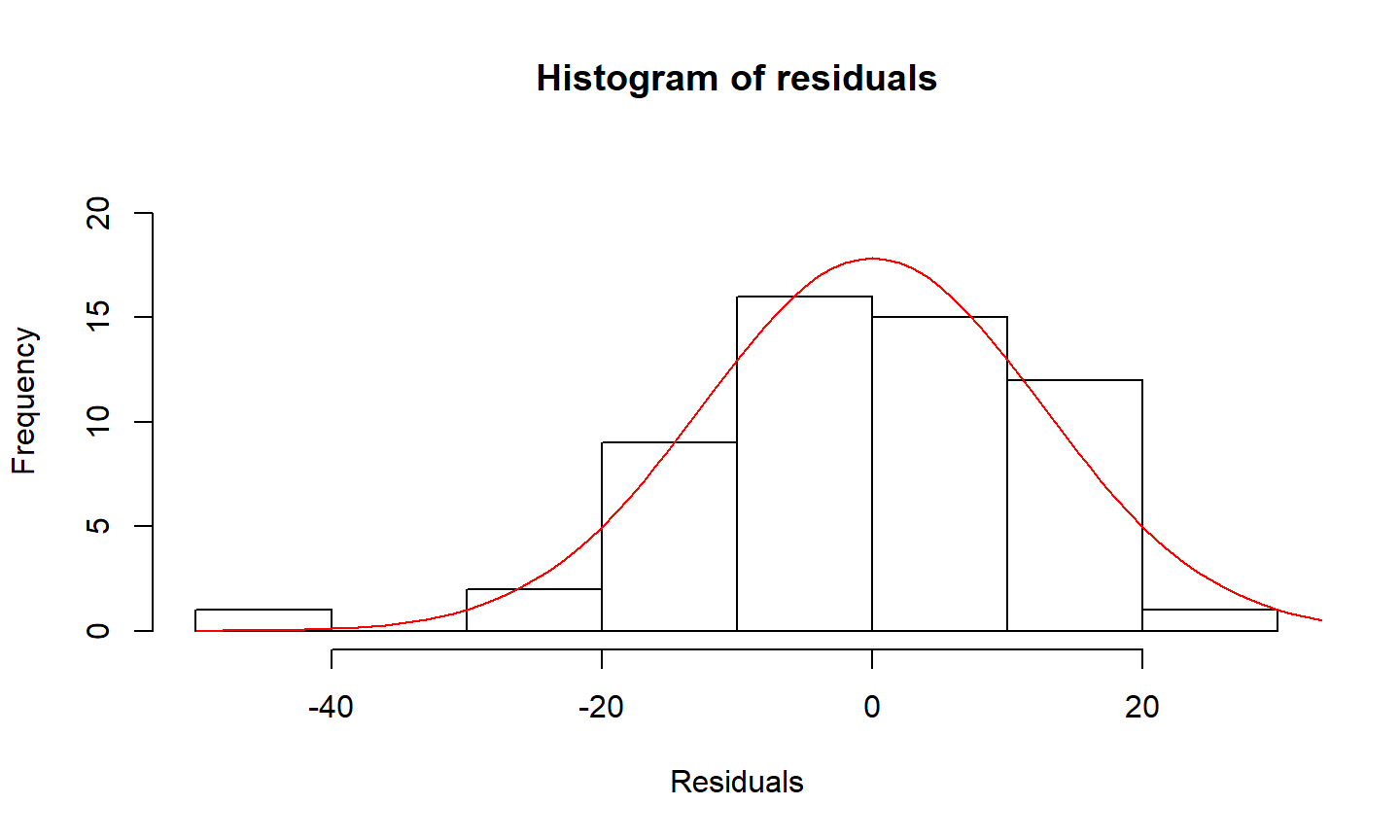In this case, the residuals seem to be slightly negatively skewed, although that is probably due to the outlier. ## Matrix algebra The multiple regression model can be written as $y_{i} = \beta_{0} + \beta_{1} x_{1,i} + \beta_{2} x_{2,i} + \cdots + \beta_{k} x_{k,i} + e_{i}.$ This expresses the relationship between a single value of the forecast variable and the predictors. Let $$\pmb{\beta} = (\beta_{0},\dots,\beta_{k})'$$ and $\pmb{Y} = \left[\begin{matrix} y_{1}\\ y_{2}\\ \vdots\\ y_{N} \end{matrix}\right] \quad \pmb{X} = \left[\begin{matrix} 1 & x_{1,1} & x_{2,1} & \dots & x_{k,1}\\ 1 & x_{1,2} & x_{2,2} & \dots & x_{k,2}\\ \vdots & \vdots & \vdots & & \vdots\\ 1 & x_{1,N} & x_{2,N} & \dots & x_{k,N} \end{matrix}\right] \quad \pmb{y} = \left[\begin{matrix} e_{1}\\ e_{2}\\ \vdots\\ e_{N} \end{matrix}\right].$ Then $\pmb{Y} = \pmb{X}\pmb{\beta} + \pmb{e}.$ ## Least squares estimation Least squares estimation is obtained by minimizing the expression $$(\pmb{Y} - \pmb{X}\pmb{\beta})'(\pmb{Y} - \pmb{X}\pmb{\beta})$$. It can be shown that this is minimized when $$\pmb{\beta}$$ takes the value $\hat{\pmb{\beta}} = (\pmb{X}'\pmb{X})^{-1}\pmb{X}'\pmb{Y}$ The estimated coefficients require the inversion of the matrix $$\pmb{X}'\pmb{X}$$. If this matrix is singular, then the model cannot be estimated. The residual variance is estimated using $\hat{\sigma}^2 = \frac{1}{N-k}(\pmb{Y} - \pmb{X}\hat{\pmb{\beta}})'(\pmb{Y} - \pmb{X}\hat{\pmb{\beta}}).$ ## Fitted values and cross-validation The normal equation shows that the fitted values can be calculated using $\pmb{\hat{Y}} = \pmb{X}\hat{\pmb{\beta}} = \pmb{X}(\pmb{X}'\pmb{X})^{-1}\pmb{X}'\pmb{Y} = \pmb{H}\pmb{Y},$ where $$\pmb{H} = \pmb{X}(\pmb{X}'\pmb{X})^{-1}\pmb{X}'$$ is known as the hat-matrix because it is used to compute $$\pmb{\hat{Y}}$$ (Y-hat). If the diagonal values of $$\pmb{H}$$ are denoted by $$h_{1},\dots,h_{N}$$, then the cross-validation statistic can be computed using $\text{CV} = \frac{1}{N}\sum_{i=1}^N [e_{i}/(1-h_{i})]^2,$ where $$e_{i}$$ is the residual obtained from fitting the model to all $$n$$ observations. ## Forecasts Let $$\pmb{X}^*$$ be a row vector containing the values of the predictors for the forecasts. Then the forecast is given by $\hat{y} = \pmb{X}^*\hat{\pmb{\beta}} = \pmb{X}^*(\pmb{X}'\pmb{X})^{-1}\pmb{X}'\pmb{Y}$ and its variance by $\sigma^2 \left[1 + \pmb{X}^* (\pmb{X}'\pmb{X})^{-1} (\pmb{X}^*)'\right].$ Then a 95% prediction interval can be calculated (assuming normally distributed errors) as $$\hat{y} \pm 1.96 \hat{\sigma} \sqrt{1 + \pmb{X}^* (\pmb{X}'\pmb{X})^{-1} (\pmb{X}^*)'}.$$ This takes account of the uncertainty due to the error term $$e$$ and the uncertainty in the coefficient estimates, but it ignores any errors in $$\pmb{X}^*$$. ## Nonlinear regression Sometimes the relationship between the forecast variable and a predictor is not linear, and then the usual multiple regression equation needs modifying. We discussed log transformations and a piecewise-linear trend in a model. Allowing other variables to enter in a nonlinear manner can be handled similarly. To keep things simple, suppose we have only one predictor $$x$$: $y=f(x)+e.$ In standard (linear) regression, $$f(x)=Î²_0+Î²_1x$$, but in nonlinear regression, we allow f to be a nonlinear function of $$x$$. One of the simplest ways to do nonlinear regression is to make $$f$$ piecewise linear. That is, we introduce points where the slope of $$f$$ can change. These points are called knots. ## Car emissions continued The relationship between carbon footprint of a car from and its city-based fuel economy is nonlinear. A change in slope occurs at about 25mpg. This can be achieved using the following variables: x (the City mpg) and $z = (x-25)_{+} = \begin{cases} 0 & \text{if } x < 25 \\ x-25 & \text{if } x\ge 25 \end{cases}$ Cityp <- pmax(fuel$City-25,0)
fit2 <- lm(Carbon ~ City + Cityp, data=fuel)
x <- 15:50; z <- pmax(x-25,0)
fcast2 <- forecast(fit2, newdata=data.frame(City=x,Cityp=z))
plot(jitter(Carbon) ~ jitter(City), data=fuel)
lines(x, fcast2$mean,col="red")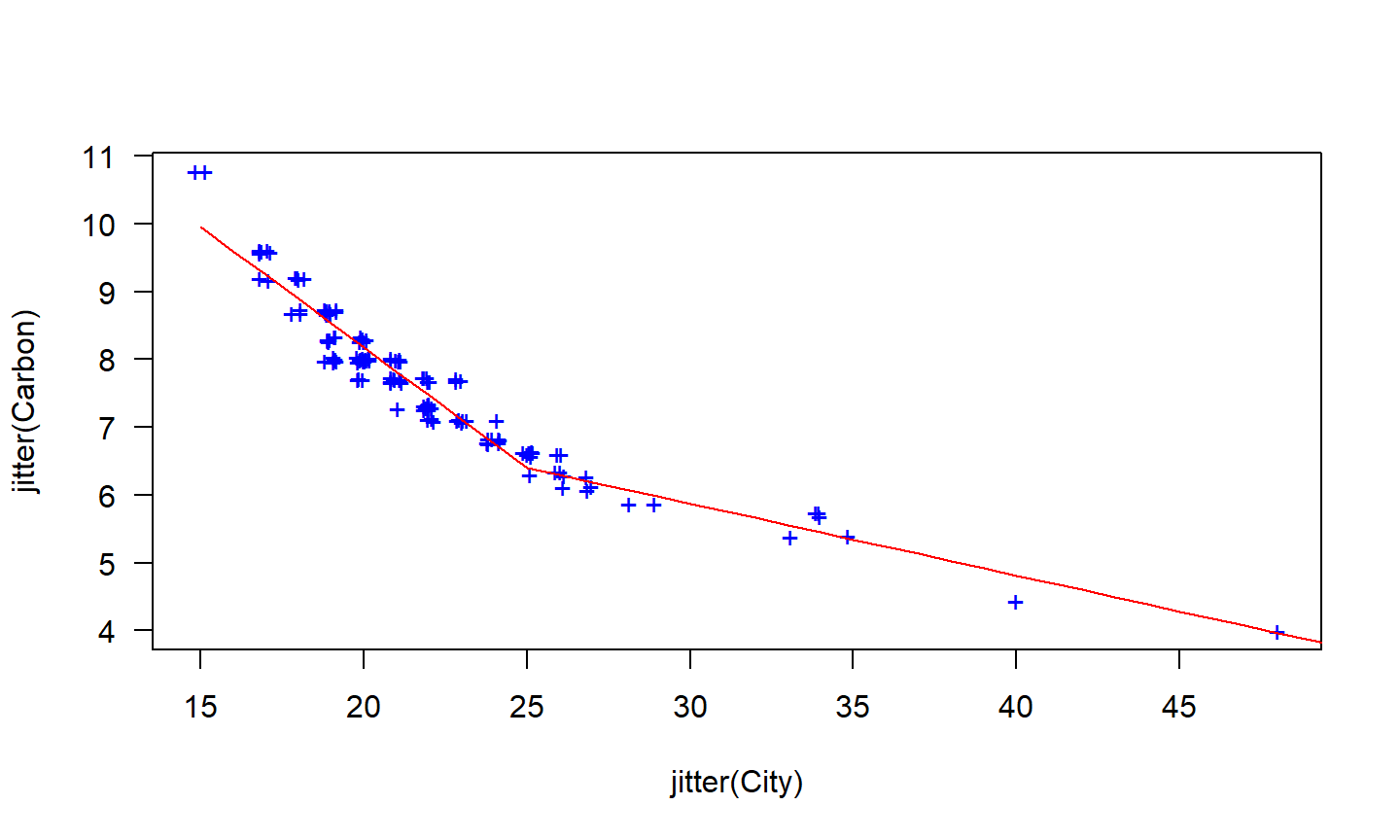Additional bends can be included in the relationship by adding further variables of the form $$(x-25)_{+}$$ where $$c$$ is the knot or point at which the line should bend. ## Regression splines Piecewise linear relationships constructed in this way are a special case of regression splines. A smoother result is obtained using piecewise cubics rather than piecewise lines. These are constrained so they are continuous (they join up) and they are smooth (so there are no sudden changes of direction as we see with piecewise linear splines). In general, a cubic regression spline is written as $x_{1}= x \ x_{2}=x^2 \ x_3=x^3 \ x_4 = (x-c_{1})^3_+ \ \dots\ x_{k} = (x-c_{k-3})^3_+.$ fit3 <- lm(Carbon ~ City + I(City^2) + I(City^3) + I(Cityp^3), data=fuel) fcast3 <- forecast(fit3,newdata=data.frame(City=x,Cityp=z)) plot(jitter(Carbon) ~ jitter(City), data=fuel) lines(x, fcast3$mean,col="red")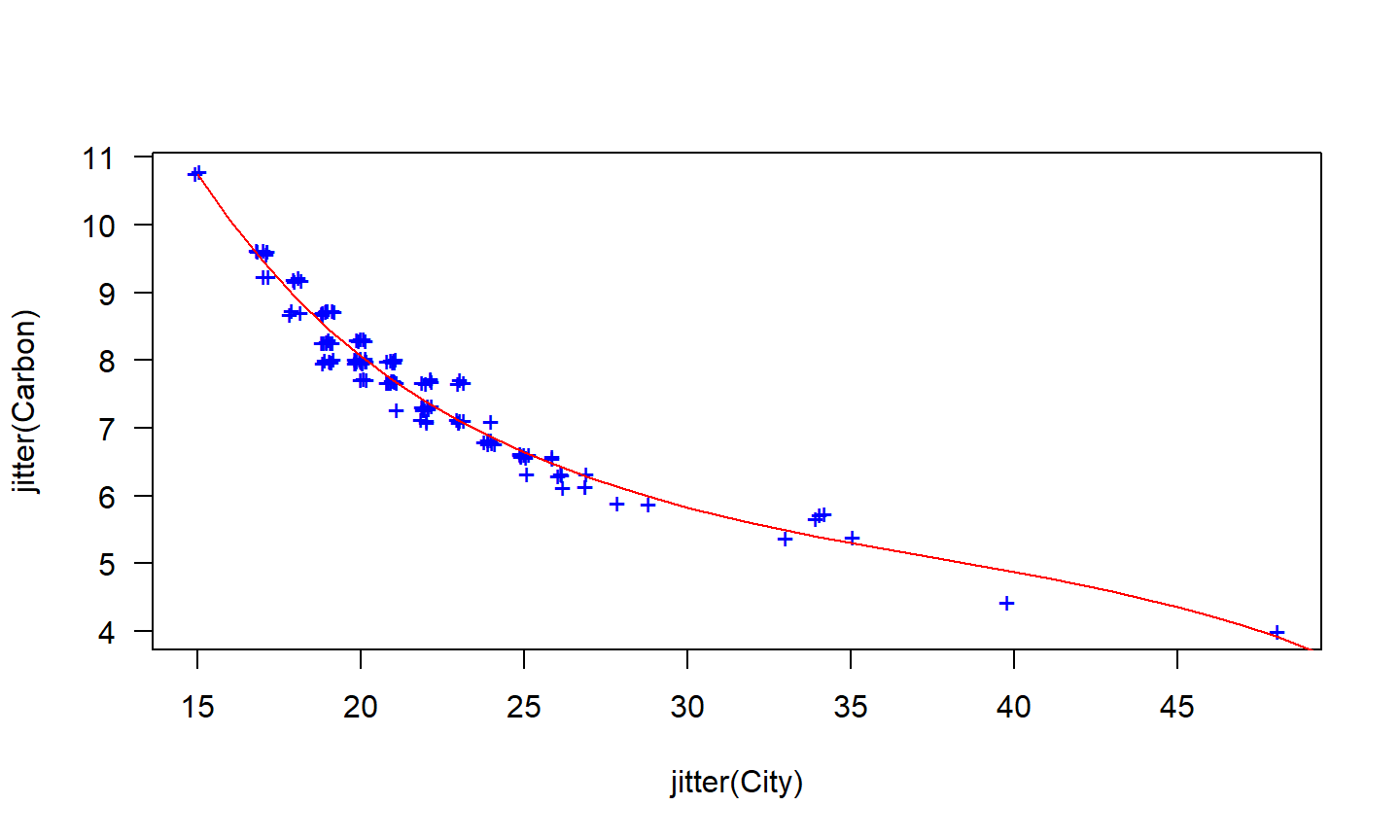This usually gives a better fit to the data, although forecasting values of Carbon when City is outside the range of the historical data becomes very unreliable.

## Correlation is not causation

A variable $$x$$ may be useful for predicting a variable $$y$$, but that does not mean $$x$$ is causing $$y$$.

For example, it is possible to model the number of drownings at a beach resort each month with the number of ice-creams sold in the same period. The two variables (ice-cream sales and drownings) are correlated (WHY?), but one is not causing the other. It is important to understand that correlations are useful for forecasting, even when there is no causal relationship between the two variables.

However, often a better model is possible if a causal mechanism can be determined. CAN YOU GIVE AN EXAMPLE?

## Confounded predictors

A related issue involves confounding variables. We say two variables are confounded when their effects on the forecast variable cannot be separated. Any pair of correlated predictors will have some level of confounding, but we would not normally describe them as confounded unless there was a relatively high level of correlation between them.

Confounding is not really a problem for forecasting, as we can still compute forecasts without needing to separate out the effects of the predictors. However, it becomes a problem with scenario forecasting as the scenarios should take account of the relationships between predictors. It is also a problem if some historical analysis of the contributions of various predictors is required.

## Multicollinearity and forecasting

A closely related issue is multicollinearity which occurs when similar information is provided by two or more of the predictor variables in a multiple regression. It can occur in a number of ways.

• Two predictors are highly correlated with each other. In this case, knowing the value of one of the variables tells you a lot about the value of the other variable.
• A linear combination of predictors is highly correlated with another linear combination of predictors. In this case, knowing the value of the first group of predictors tells you a lot about the value of the second group of predictors.

Suppose you have quarterly data and use four dummy variables, $$D_1,D_2,D_3$$ and $$D_4$$. Then $$D_4=1 - D_1 - D_2 - D_3$$, so there is perfect correlation between $$D_4$$ and $$D_1+D_2+D_3$$.

## Multicollinearity and forecasting

When multicollinearity occurs in a multiple regression model, there are several consequences that you need to be aware of.

• If there is perfect correlation, it is not possible to estimate the regression model.
• If there is high correlation, then the estimation of the regression coefficients is computationally difficult.
• The uncertainty associated with individual regression coefficients will be large. This is because they are difficult to estimate. Consequently, statistical tests (e.g., t-tests) on regression coefficients are unreliable. Also, it will not be possible to make accurate statements about the contribution of each separate predictor to the forecast.
• Forecasts will be unreliable if the values of the future predictors are outside the range of the historical values of the predictors.

Otherwise, multicollinearity is not a problem for forecasting.

## What have we learned today?

• Recap multiple regression
• Fit, forecast, residuals in multiple regressions
• Seasonal dummies
• Trend
• Residuals and residual autocorrelation
• Regressions in matrix form (H hat matrix)
• Nonlinear regressions and splides
• Correlation and causation